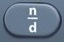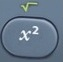Basics
A quadratic is an equation or formula in which a variable is squared.
(In the equation above, the variable x is squared.)

A question involving quadratics could involve a relatively simple plug-in, where you plug in a number for x to solve for y using the quadratic equation.

A question involving quadratics could involve more complicated calculations, where you must solve for x using the quadratic formula.

Because the quadratic formula features ± calculations, in most cases you will produce two answers for x.

When squaring a negative number, remember to use parentheses to protect the order of operations.

Formulas (Mathematics Formula Sheet)
standard form of a quadratic equation
y =$\bf\displaystyle\ a{{x}^{2}}+bx+c$

x =$\bf\displaystyle\frac{-b\pm \sqrt{{{b}^{2}}-4ac}}{2a}$

Formulas (NOT on Mathematics Formula Sheet)

x =$\bf\displaystyle\frac{-b\pm \sqrt{{{b}^{2}}-4ac}}{2a}$ means  —>$\bf\displaystyle x=\frac{-b+\sqrt{{{b}^{2}}-4ac}}{2a}$

OR$\bf\displaystyle x=\frac{-b-\sqrt{{{b}^{2}}-4ac}}{2a}$

Question
For the quadratic equation y = 4x² – 18x + 20, solve for y when x = 3.

y = 2

See Plug-In.
y = 4x² – 18x + 20
y = 4 × 3² – 18 × 3 + 20 = 2

Input Display Commentblinker clears screen
4 × 3– 18 × 3 + 20 4*3²-18*3+20 y = 4x² – 18x + 20
Plug in 3 for x.2 Answer

Question
For the quadratic equation y = 4x² – 18x + 20, solve for y when x = -3.

y = 110

See Plug-In.
y = 4x² – 18x + 20
y = 4 × (-3)² – 18 × -3 + 20 = 110

Input Display Commentblinker clears screen
4 ×-3– 18 × -3 + 20
4*(-3)²-18*-3+20 y = 4x² – 18x + 20
Plug in -3 for x.

###### Parentheses protect order of operations.110 Answer

Question
For the quadratic equation 2x² – 9x + 4 = 0, solve for x.

x =$\bf\displaystyle\frac{1}{2}$  and  x = 4

x =$\bf\displaystyle\frac{-b\pm \sqrt{{{b}^{2}}-4ac}}{2a}$

ax² + bx + c = 0
2x² – 9x + 4 = 0
a = 2
b = -9
c = 4$\bf\displaystyle x=\frac{-b+\sqrt{{{b}^{2}}-4ac}}{2a}$$\bf\displaystyle x=\frac{--9+\sqrt{{{(-9)}^{2}}-4\times 2\times 4}}{2\times 2}$  = 4

OR$\bf\displaystyle x=\frac{-b-\sqrt{{{b}^{2}}-4ac}}{2a}$$\bf\displaystyle x=\frac{--9-\sqrt{{{(-9)}^{2}}-4\times 2\times 4}}{2\times 2}$  =$\bf\displaystyle\frac{1}{2}$

Input Display Commentblinker clears screen– -9 +-9– 4 × 2 × 42 × 2$\bf\displaystyle x=\frac{--9+\sqrt{{{(-9)}^{2}}-4\times 2\times 4}}{2\times 2}$$\bf\displaystyle x=\frac{-b+\sqrt{{{b}^{2}}-4ac}}{2a}$4 Answer– -9 –-9– 4 × 2 × 42 × 2$\bf\displaystyle x=\frac{--9-\sqrt{{{(-9)}^{2}}-4\times 2\times 4}}{2\times 2}$$\bf\displaystyle x=\frac{-b-\sqrt{{{b}^{2}}-4ac}}{2a}$$\bf\displaystyle\frac{1}{2}$ Answer

Practice – Questions
1.  For the quadratic equation y = x² + 6x + 8, solve for y when x = 2.

2.  For the quadratic equation y = x² + 6x + 8, solve for y when x = -2.

3.  For the quadratic equation y = 6x² – 18x + 12, solve for y when x = 4.

4.  For the quadratic equation y = 6x² – 18x + 12, solve for y when x = -4.

5.  For the quadratic equation 3x² – 7x + 4 = 0, solve for x.

5.  x = 1  or  x =$\bf\displaystyle\frac{4}{3}$